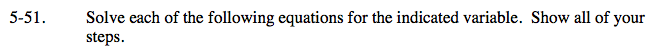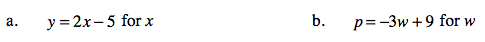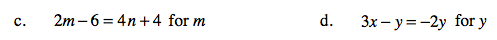Home > AC > Chapter 5 > Lesson 5.1.5 > Problem5-51

5-51.
1. Solve each of the following equations for the indicated variable. Show all of your steps. Homework Help ✎

1. y = 2x − 5 for x

2. p = −3w + 9 for w

3. 2m − 6 = 4n + 4 for m

4. 3xy = −2y for yIsolate the variable on one side.

y = 2x − 5
+5 + 5
y + 5 = 2x

Solve for x.

$\frac{y+5}{2}=x$

Follow the steps in part (a).Follow the steps in part (a).

m = 2n +5

Follow the steps in part (a).

y = −3x